# pylops.LinearOperator#

class pylops.LinearOperator(Op=None, dtype=None, shape=None, dims=None, dimsd=None, clinear=None, explicit=None, name=None)[source]#

Common interface for performing matrix-vector products.

This class acts as an abstract interface between matrix-like objects and iterative solvers, providing methods to perform matrix-vector and adjoint matrix-vector products as as well as convenience methods such as eigs, cond, and conj.

Note

End users of PyLops should not use this class directly but simply use operators that are already implemented. This class is meant for developers and it has to be used as the parent class of any new operator developed within PyLops. Find more details regarding implementation of new operators at Implementing new operators.

Parameters
Op

Operator. If other arguments are provided, they will overwrite those obtained from Op.

dtypestr, optional

Type of elements in input array.

shapetuple(int, int), optional

Shape of operator. If not provided, obtained from dims and dimsd.

dimstuple(int, ..., int), optional

New in version 2.0.0.

Dimensions of model. If not provided, (self.shape,) is used.

dimsdtuple(int, ..., int), optional

New in version 2.0.0.

Dimensions of data. If not provided, (self.shape,) is used.

explicitbool, optional

Operator contains a matrix that can be solved explicitly (True) or not (False). Defaults to False.

clinearbool, optional

New in version 1.17.0.

Operator is complex-linear. Defaults to True.

namestr, optional

New in version 2.0.0.

Name of operator (to be used by pylops.utils.describe.describe)

Methods

 __init__([Op, dtype, shape, dims, dimsd, ...]) adjoint() apply_columns(cols) Apply subset of columns of operator cond([uselobpcg]) Condition number of linear operator. conj() Complex conjugate operator div(y[, niter, densesolver]) Solve the linear problem $$\mathbf{y}=\mathbf{A}\mathbf{x}$$. dot(x) Matrix-matrix or matrix-vector multiplication. eigs([neigs, symmetric, niter, uselobpcg]) Most significant eigenvalues of linear operator. matmat(X) Matrix-matrix multiplication. matvec(x) Matrix-vector multiplication. reset_count() Reset counters rmatmat(X) Matrix-matrix multiplication. rmatvec(x) Adjoint matrix-vector multiplication. todense([backend]) Return dense matrix. toimag([forw, adj]) Imag operator toreal([forw, adj]) Real operator tosparse() Return sparse matrix. trace([neval, method, backend]) Trace of linear operator. transpose()

## Examples using pylops.LinearOperator#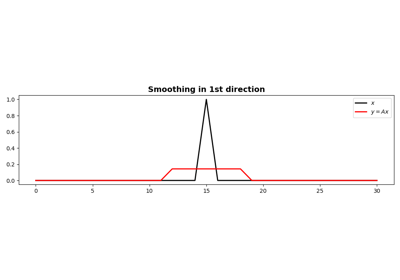1D Smoothing

1D Smoothing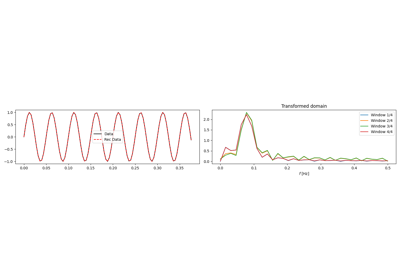1D, 2D and 3D Sliding

1D, 2D and 3D Sliding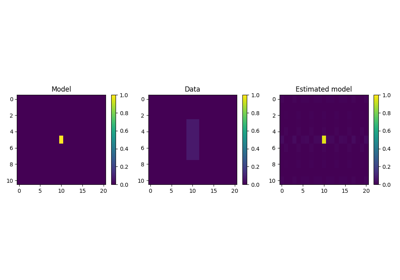2D Smoothing

2D Smoothing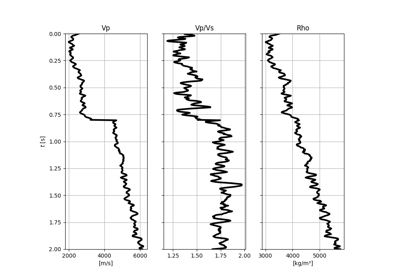AVO modelling

AVO modelling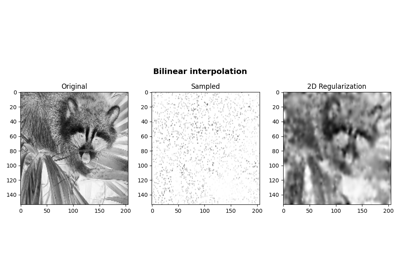Bilinear Interpolation

Bilinear Interpolation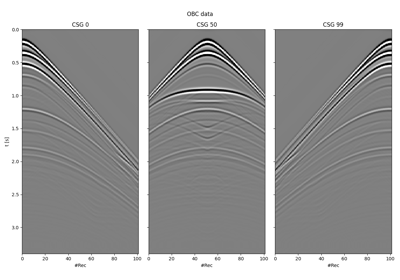Blending

Blending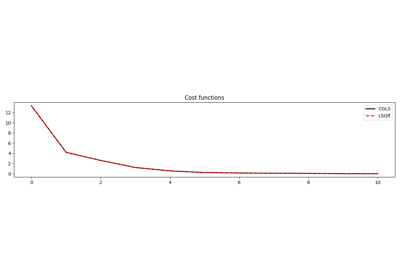CGLS and LSQR Solvers

CGLS and LSQR Solvers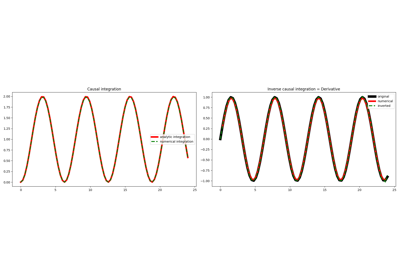Causal Integration

Causal Integration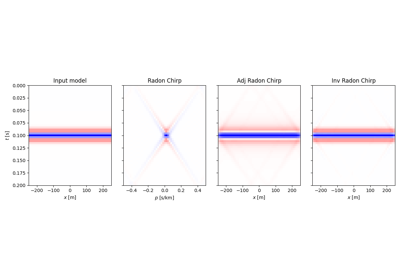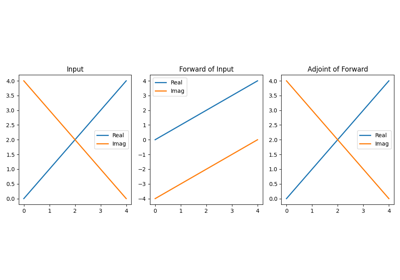Conj

Conj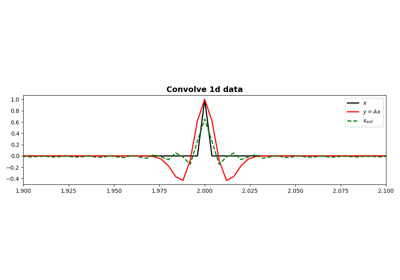Convolution

Convolution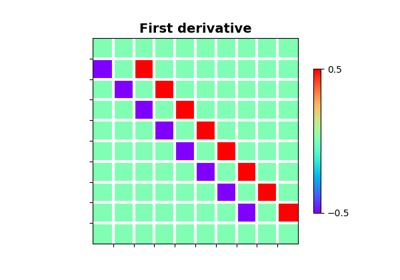Derivatives

DerivativesDescribe

Describe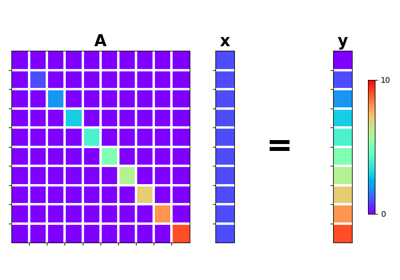Diagonal

Diagonal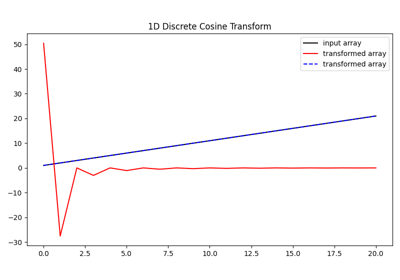Discrete Cosine Transform

Discrete Cosine Transform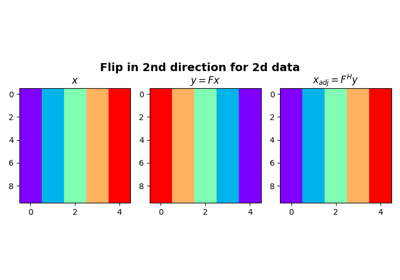Flip along an axis

Flip along an axis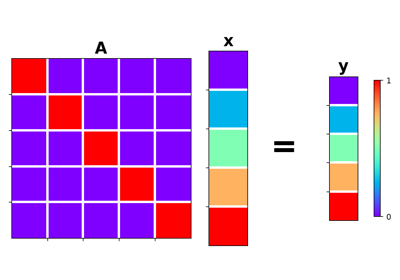Identity

Identity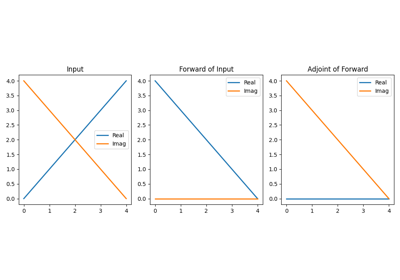Imag

Imag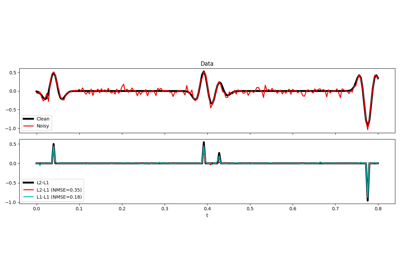L1-L1 IRLS

L1-L1 IRLS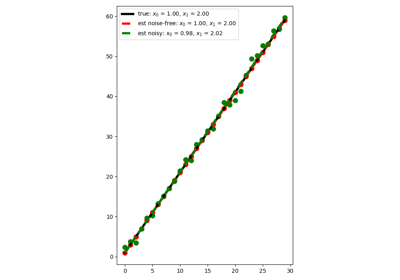Linear Regression

Linear Regression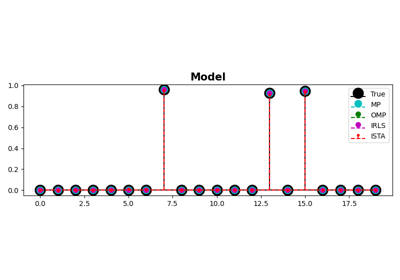MP, OMP, ISTA and FISTA

MP, OMP, ISTA and FISTA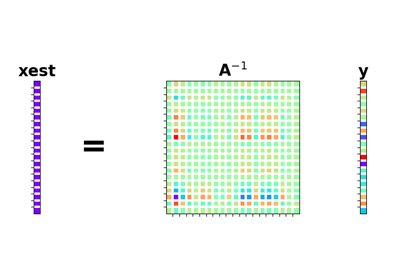Matrix Multiplication

Matrix Multiplication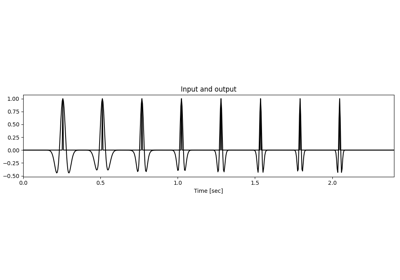Non-stationary Convolution

Non-stationary Convolution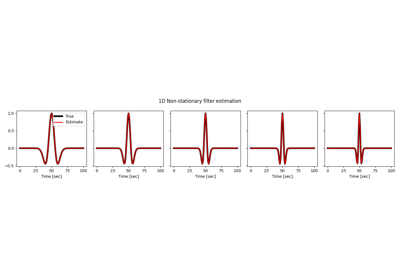Non-stationary Filter Estimation

Non-stationary Filter EstimationNormal Moveout (NMO) Correction

Normal Moveout (NMO) Correction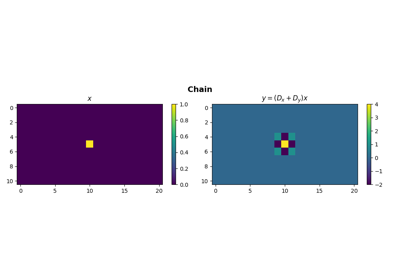Operators concatenation

Operators concatenation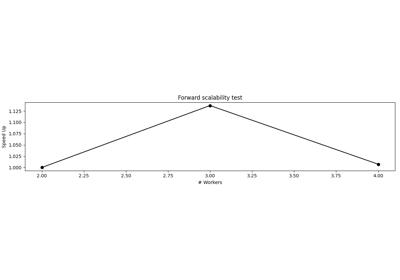Operators with Multiprocessing

Operators with Multiprocessing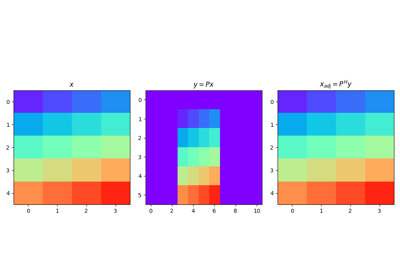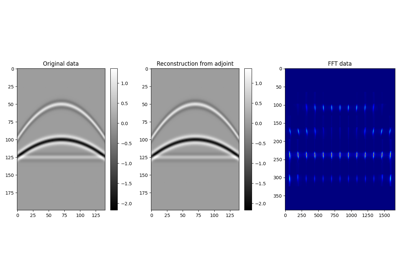Patching

Patching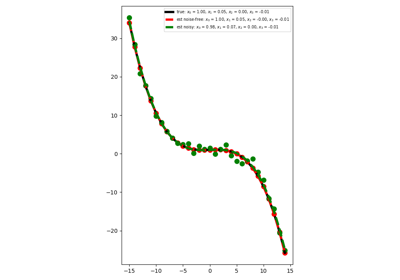Polynomial Regression

Polynomial Regression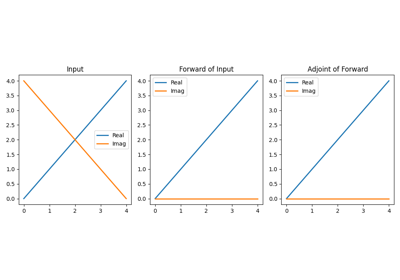Real

Real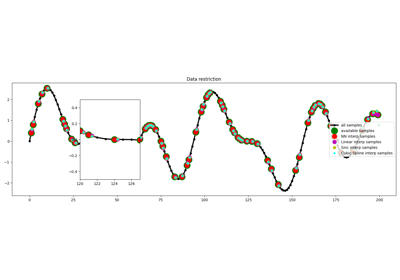Restriction and Interpolation

Restriction and Interpolation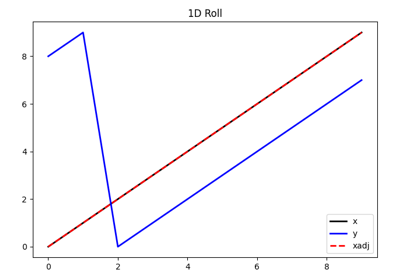Roll

Roll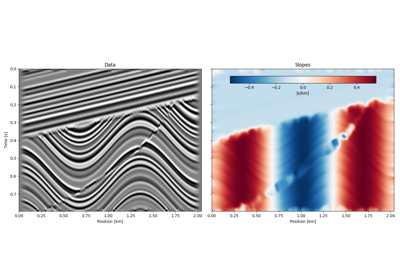Seislet transform

Seislet transform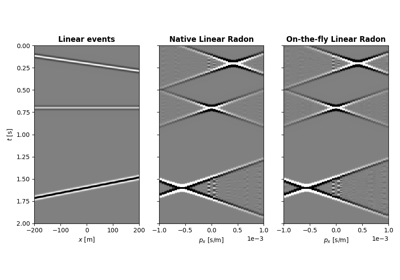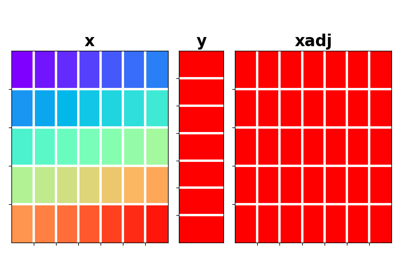Sum

Sum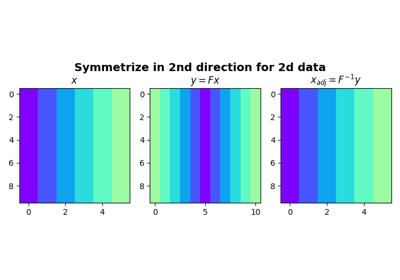Symmetrize

Symmetrize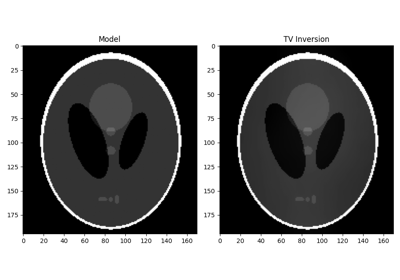Total Variation (TV) Regularization

Total Variation (TV) Regularization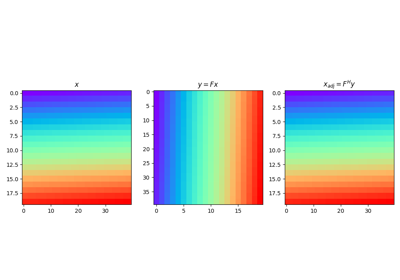Transpose

Transpose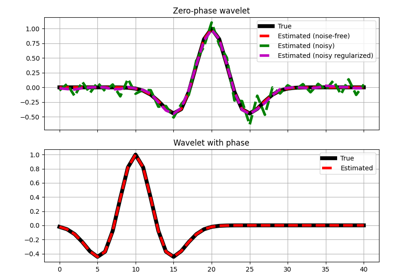Wavelet estimation

Wavelet estimation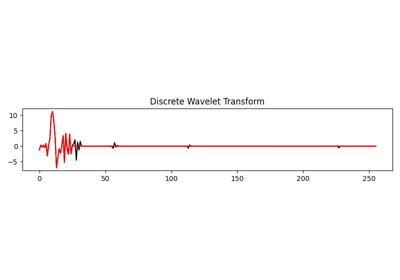Wavelet transform

Wavelet transform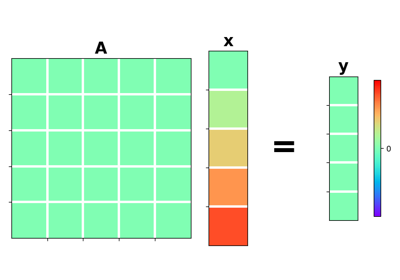Zero

Zero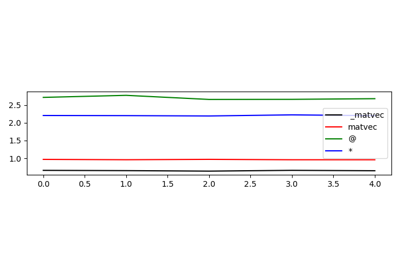01. The LinearOperator

01. The LinearOperator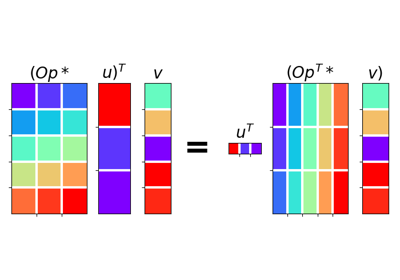02. The Dot-Test

02. The Dot-Test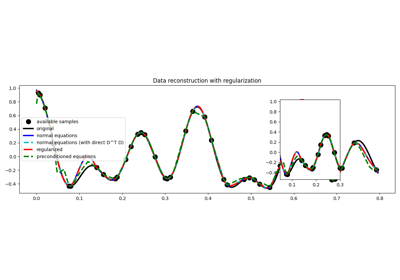03. Solvers

03. Solvers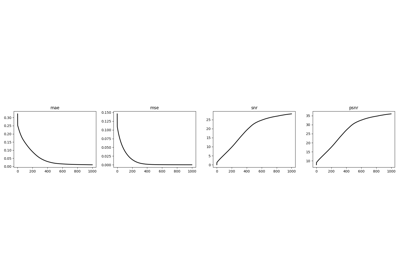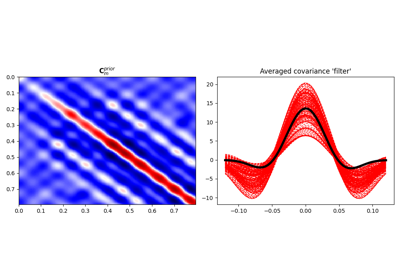04. Bayesian Inversion

04. Bayesian Inversion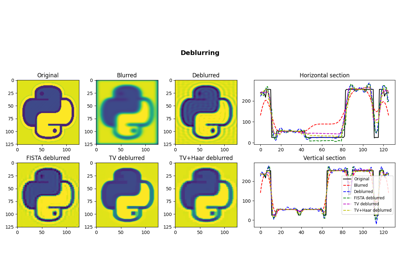05. Image deblurring

05. Image deblurring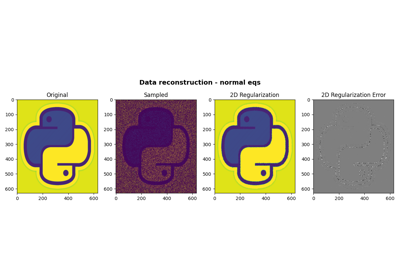06. 2D Interpolation

06. 2D Interpolation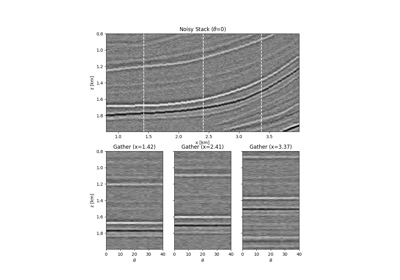08. Pre-stack (AVO) inversion

08. Pre-stack (AVO) inversion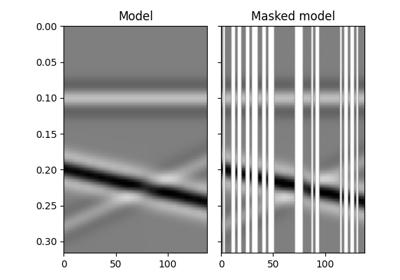12. Seismic regularization

12. Seismic regularization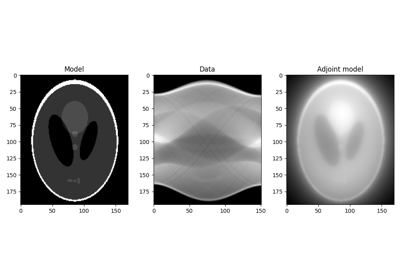16. CT Scan Imaging

16. CT Scan Imaging17. Real/Complex Inversion

17. Real/Complex Inversion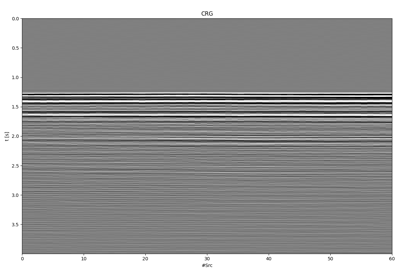18. Deblending

18. Deblending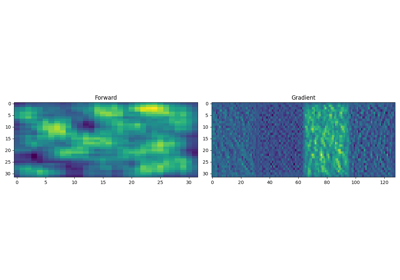19. Automatic Differentiation

19. Automatic Differentiation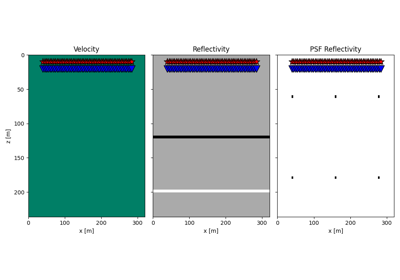20. Image Domain Least-squares migration

20. Image Domain Least-squares migration